Created December 30, 2019 © CC BY-NC

# Ada Robot Car With Neural Network

With this smart robot, we will use a neural network to follow the light and stop when it reaches its goal.

AdvancedFull instructions provided24 hours251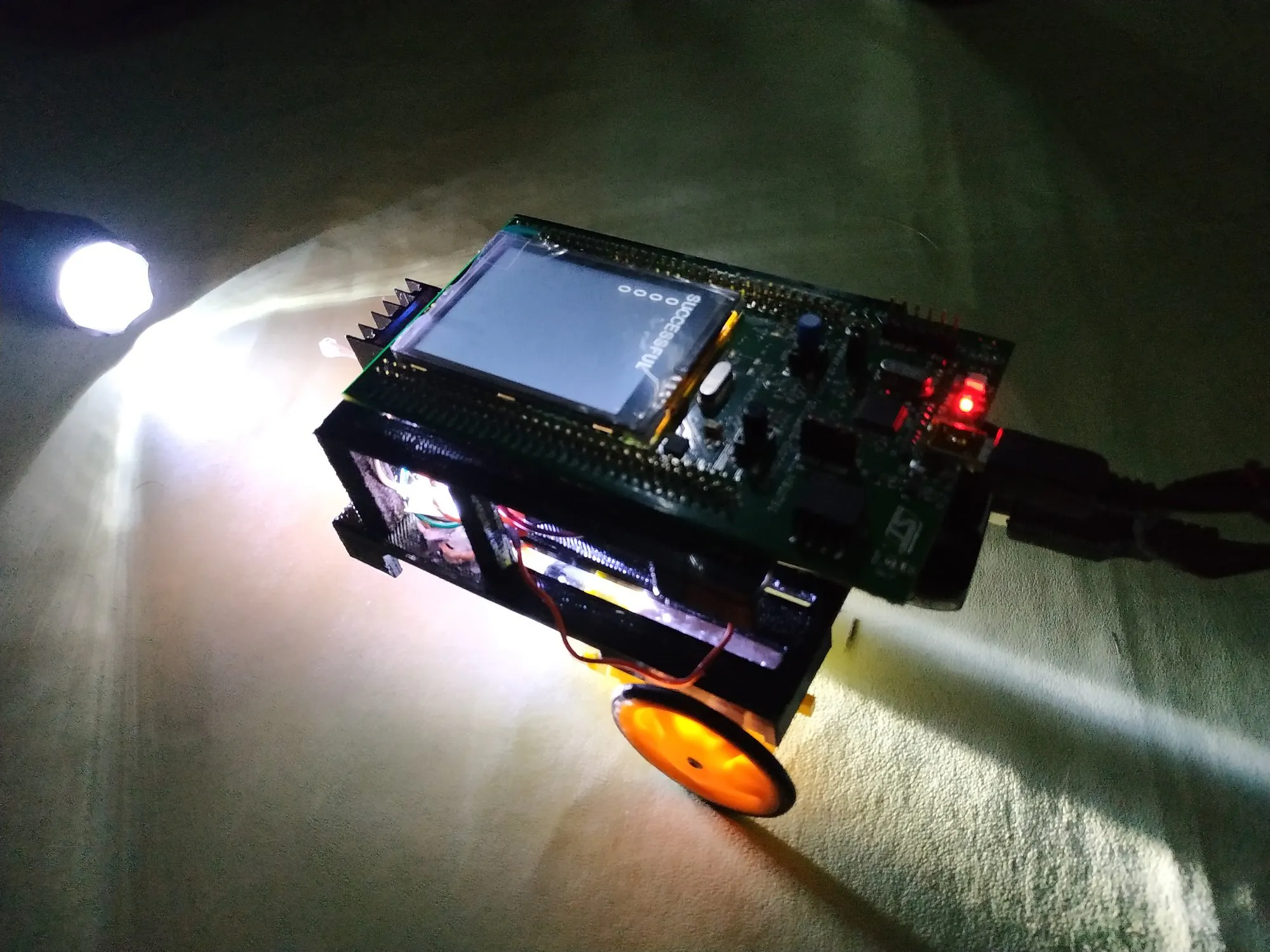## Things used in this project

### Hardware components

 STM32F429I-DISC1
×1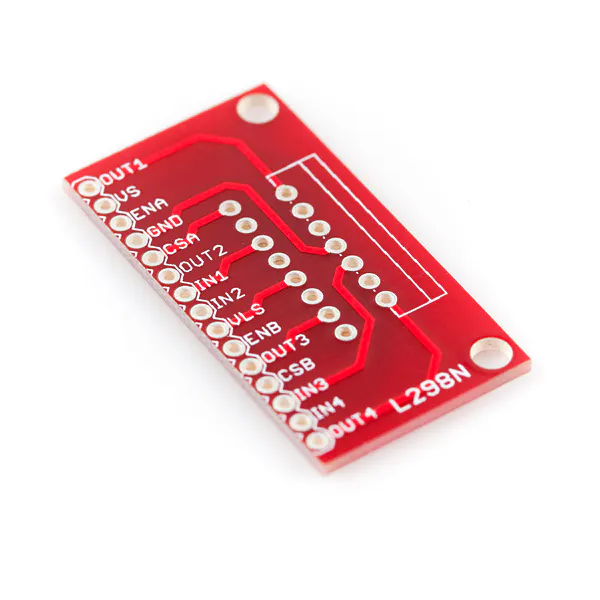SparkFun Full-Bridge Motor Driver Breakout - L298N
×1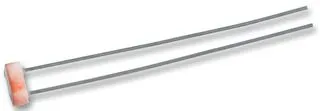LDR, 5 Mohm
×1
 Gearmotor (generic)
×2Resistor 10k ohm
×3
 Ball caster
×1
 Rechearchable battery 5V 2A
×1

### Software apps and online servicesAdaCore GNAT Community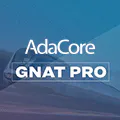AdaCore GNAT Pro
 Python 3.7.3

### Hand tools and fabrication machines3D Printer (generic)
 Lamp

Read more

## Custom parts and enclosures

### microrobot-chassis-adacore

STL, FCStd and JPG files of a Ada Robot Car With Neural Network

## Schematics

### Electric diagram

Electrical connections of the robot car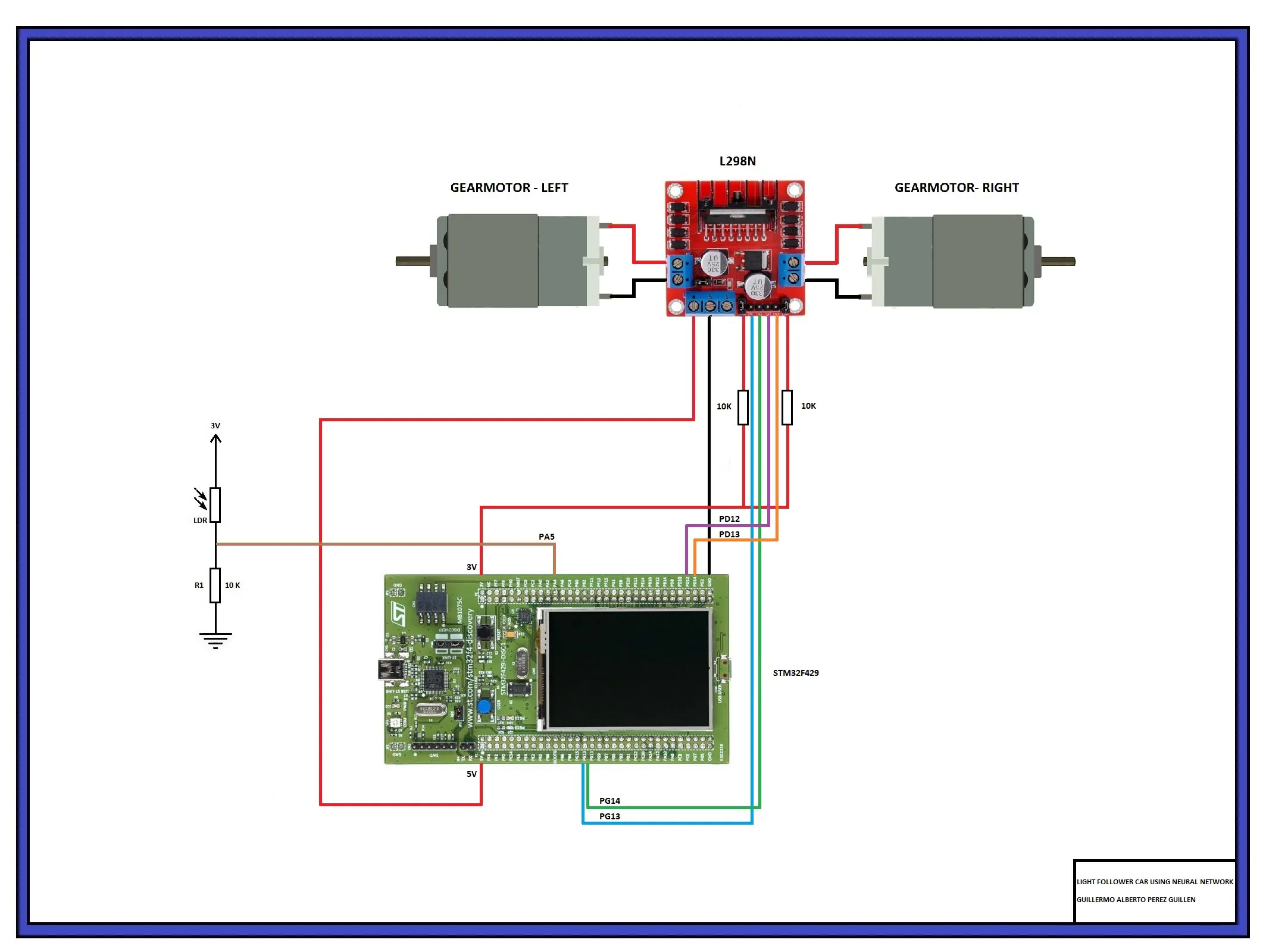## Code

### autonomous_ada_car.adb

ADA
Developed with GNAT Programming Studio. This code must be compiled and uploaded to the STM32F429I board
```-- AUTHOR: GUILLERMO ALBERETO PEREZ GUILLEN

with Last_Chance_Handler;  pragma Unreferenced (Last_Chance_Handler);

with STM32.Board;  use STM32.Board;
with STM32.Device; use STM32.Device;

with HAL;          use HAL;
with STM32.ADC;    use STM32.ADC;
with STM32.GPIO;   use STM32.GPIO;

with LCD_Std_Out;

with Ada.Real_Time;  use Ada.Real_Time;

with Ada.Numerics; use Ada.Numerics;

with Ada.Numerics.Elementary_Functions;
use  Ada.Numerics.Elementary_Functions;

procedure Autonomous_Ada_Car is

Converter     : Analog_To_Digital_Converter renames ADC_1;
Input_Channel : constant Analog_Input_Channel := 5;
Input         : constant GPIO_Point := PA5;

LED1       : GPIO_Point renames PG13; -- GREEN LED
LED2       : GPIO_Point renames PG14; -- RED LED
LED3       : GPIO_Point renames PD12;
LED4       : GPIO_Point renames PD13;

--  See the mapping of channels to GPIO pins at the top of the ADC package.
--  Also see the board's User Manual for which GPIO pins are available.
--  For example, on the F429 Discovery board, PA5 is not used by some   --  other device, and maps to ADC channel 5.

All_Regular_Conversions : constant Regular_Channel_Conversions :=
(1 => (Channel => Input_Channel, Sample_Time => Sample_144_Cycles));

Raw : UInt32 := 0;
light_Current : Integer;
light_Before : Integer;
eval : Float;
lux : Float;

HiddenWeights_1_1, HiddenWeights_1_2, HiddenWeights_1_3, HiddenWeights_1_4 : Float;
HiddenWeights_2_1, HiddenWeights_2_2, HiddenWeights_2_3, HiddenWeights_2_4 : Float;
HiddenWeights_3_1, HiddenWeights_3_2, HiddenWeights_3_3, HiddenWeights_3_4 : Float;

OutputWeights_1_1, OutputWeights_1_2, OutputWeights_1_3, OutputWeights_1_4 : Float;
OutputWeights_2_1, OutputWeights_2_2, OutputWeights_2_3, OutputWeights_2_4 : Float;
OutputWeights_3_1, OutputWeights_3_2, OutputWeights_3_3, OutputWeights_3_4 : Float;
OutputWeights_4_1, OutputWeights_4_2, OutputWeights_4_3, OutputWeights_4_4 : Float;

Hidden_Layer_1_1, Hidden_Layer_1_2, Hidden_Layer_1_3, Hidden_Layer_1_4 : Float;
Output_Layer_1_1, Output_Layer_1_2, Output_Layer_1_3, Output_Layer_1_4 : Float;

Output_1, Output_2, Output_3, Output_4 : Integer;

Successful : Boolean;

procedure Print (X, Y : Natural; Value : String);

procedure Configure_Analog_Input;

-----------
-- LCD Print --
-----------

procedure Print (X, Y : Natural; Value : String) is
Trailing_Blanks : constant String := "   ";  -- to clear the rest of line
begin
--LCD_Std_Out.Put (X, Y, Value & " / 4095" & Trailing_Blanks);
LCD_Std_Out.Put (X, Y, Value & Trailing_Blanks);
end Print;

----------------------------
-- Configure_Analog_Input --
----------------------------

procedure Configure_Analog_Input is
begin
Enable_Clock (Input);
Configure_IO (Input, (Mode => Mode_Analog, Resistors => Floating));
end Configure_Analog_Input;

begin

Initialize_LEDs;
LED1.Clear;
LED2.Clear;
LED3.Clear;
LED4.Clear;

Configure_Analog_Input;

Enable_Clock (Converter);

Reset_All_ADC_Units;

Configure_Common_Properties
(Mode           => Independent,
Prescalar      => PCLK2_Div_2,
DMA_Mode       => Disabled,
Sampling_Delay => Sampling_Delay_5_Cycles);

Configure_Unit
(Converter,
Resolution => ADC_Resolution_12_Bits,
Alignment  => Right_Aligned);

Configure_Regular_Conversions
(Converter,
Continuous  => False,
Trigger     => Software_Triggered,
Enable_EOC  => True,
Conversions => All_Regular_Conversions);

Enable (Converter);

light_Current := 0;
light_Before := 0;

-------------------------------------------
--- NEURONAL NETWORKS' WEIGHTS
-------------------------------------------
HiddenWeights_1_1 := -0.8330212122953609;
HiddenWeights_1_2 := 1.0912649297996564;
HiddenWeights_1_3 := -0.6179969826549335;
HiddenWeights_1_4 := -1.0762413280914194;

HiddenWeights_2_1 := -0.7221015071612642;
HiddenWeights_2_2 := -0.3040531641938827;
HiddenWeights_2_3 := 1.424273154914373;
HiddenWeights_2_4 := 0.5226012446435597;

HiddenWeights_3_1 := -1.3873042452980089;
HiddenWeights_3_2 := 0.8796185107005765;
HiddenWeights_3_3 := 0.6115239126364166;
HiddenWeights_3_4 := -0.6941384010920131;

OutputWeights_1_1 := 0.4890791000431967;
OutputWeights_1_2 := -1.2072393706400335;
OutputWeights_1_3 := -1.1170823069750404;
OutputWeights_1_4 := 0.08254392928517773;

OutputWeights_2_1 := 1.2585395954445326;
OutputWeights_2_2 := 0.7259701403757809;
OutputWeights_2_3 := 0.05232196665284013;
OutputWeights_2_4 := 0.5379573853597585;

OutputWeights_3_1 := 1.3028834913318572;
OutputWeights_3_2 := -1.3073304956402805;
OutputWeights_3_3 := 0.1681659447995217;
OutputWeights_3_4 := -0.016766185238717802;

OutputWeights_4_1 := -0.38086087439361543;
OutputWeights_4_2 := 0.8415209522385925;
OutputWeights_4_3 := -1.527573567687556;
OutputWeights_4_4 := 0.476559350936026;

loop
light_Before := light_Current;
Start_Conversion (Converter);

Poll_For_Status (Converter, Regular_Channel_Conversion_Complete, Successful);
if not Successful then
Print (0, 0, "NOT SUCCESSFUL");
else
Print (0, 0, "SUCCESSFUL"); -- Successful readings
Raw := UInt32 (Conversion_Value (Converter));
light_Current := Integer(Raw * 1);

----------------------------------------------
-- CHARGE INPUT VALUES...
----------------------------------------------
if light_Current >= light_Before then -- If light_Current is greater than or equal to, move forward
eval := 1.0;
else -- else, it turns counterclockwise
eval := -1.0;
end if;

if light_Current <= 3750 then -- If light_Current is less than, move forward or turn to the left
lux := 1.0;
else -- else, "Stop"
lux := -1.0;
end if;
----------------------------------------------
-- Input * HiddenWeights
-- We use the Tanh to get values between 1 and -1
----------------------------------------------
Hidden_Layer_1_1 := Tanh(1.0*HiddenWeights_1_1 + eval*HiddenWeights_2_1 + lux*HiddenWeights_3_1);
Hidden_Layer_1_2 := Tanh(1.0*HiddenWeights_1_2 + eval*HiddenWeights_2_2 + lux*HiddenWeights_3_2);
Hidden_Layer_1_3 := Tanh(1.0*HiddenWeights_1_3 + eval*HiddenWeights_2_3 + lux*HiddenWeights_3_3);
Hidden_Layer_1_4 := Tanh(1.0*HiddenWeights_1_4 + eval*HiddenWeights_2_4 + lux*HiddenWeights_3_4);
----------------------------------------------
-- Hidden_Layers * OutputWeights
-- We use the Tanh to get values between 1 and -1
----------------------------------------------
Output_Layer_1_1 := Tanh(Hidden_Layer_1_1*OutputWeights_1_1 + Hidden_Layer_1_2*OutputWeights_2_1 + Hidden_Layer_1_3*OutputWeights_3_1 + Hidden_Layer_1_4*OutputWeights_4_1);
Output_Layer_1_2 := Tanh(Hidden_Layer_1_1*OutputWeights_1_2 + Hidden_Layer_1_2*OutputWeights_2_2 + Hidden_Layer_1_3*OutputWeights_3_2 + Hidden_Layer_1_4*OutputWeights_4_2);
Output_Layer_1_3 := Tanh(Hidden_Layer_1_1*OutputWeights_1_3 + Hidden_Layer_1_2*OutputWeights_2_3 + Hidden_Layer_1_3*OutputWeights_3_3 + Hidden_Layer_1_4*OutputWeights_4_3);
Output_Layer_1_4 := Tanh(Hidden_Layer_1_1*OutputWeights_1_4 + Hidden_Layer_1_2*OutputWeights_2_4 + Hidden_Layer_1_3*OutputWeights_3_4 + Hidden_Layer_1_4*OutputWeights_4_4);
----------------------------------------------
-- We charge absolute and integer values at the outputs
----------------------------------------------
Output_1 := Integer (abs (Output_Layer_1_1));
Output_2 := Integer (abs (Output_Layer_1_2));
Output_3 := Integer (abs (Output_Layer_1_3));
Output_4 := Integer (abs (Output_Layer_1_4));
----------------------------------------------
-- Activate the outputs according to the calculations of the neural network
----------------------------------------------
if Output_1 = 1 then
LED1.Set;
else
LED1.Clear;
end if;

if Output_2 = 1 then
LED2.Set;
else
LED2.Clear;
end if;

if Output_3 = 1 then
LED3.Set;
else
LED3.Clear;
end if;

if Output_4 = 1 then
LED4.Set;
else
LED4.Clear;
end if;
----------------------------------------------
-- Print the outputs of the neural network
----------------------------------------------
Print (0, 25, Output_1'Img);
Print (0, 50, Output_2'Img);
Print (0, 75, Output_3'Img);
Print (0, 100, Output_4'Img);

end if;

delay until Clock + Milliseconds (100); -- slow it down to ease reading
end loop;
end Autonomous_Ada_Car;
```

### autonomous_ada_car.gpr

ADA
Project data, developed with GNAT Programming Studio
```with "../../../../../boards/stm32f429_discovery/stm32f429_discovery_full.gpr";

project Autonomous_Ada_Car extends "../../../../../examples/shared/common/common.gpr" is

for Main use ("autonomous_ada_car.adb");

for Languages use ("Ada");
for Source_Dirs use ("src");
for Object_Dir use "obj/" & STM32F429_Discovery_Full.Build;
for Runtime ("Ada") use STM32F429_Discovery_Full'Runtime("Ada");
for Create_Missing_Dirs use "true";

package Builder is
for Global_Configuration_Pragmas use "gnat.adc";
end Builder;

package Compiler renames STM32F429_Discovery_Full.Compiler;

end Autonomous_Ada_Car;
```

### NeuralNetwork

Python
Code to test our neural network and to obtain hidden weights
```import numpy as np

# We create the class
class NeuralNetwork:

def __init__(self, layers, activation='tanh'):
if activation == 'sigmoid':
self.activation = sigmoid
self.activation_prime = sigmoid_derivada
elif activation == 'tanh':
self.activation = tanh
self.activation_prime = tanh_derivada

# Initialize the weights
self.weights = []
self.deltas = []
# Assign random values to input layer and hidden layer
for i in range(1, len(layers) - 1):
r = 2*np.random.random((layers[i-1] + 1, layers[i] + 1)) -1
self.weights.append(r)
# Assigned random to output layer
r = 2*np.random.random( (layers[i] + 1, layers[i+1])) - 1
self.weights.append(r)

def fit(self, X, y, learning_rate=0.2, epochs=100000):
# I add column of ones to the X inputs. With this we add the Bias unit to the input layer
ones = np.atleast_2d(np.ones(X.shape))
X = np.concatenate((ones.T, X), axis=1)

for k in range(epochs):
i = np.random.randint(X.shape)
a = [X[i]]

for l in range(len(self.weights)):
dot_value = np.dot(a[l], self.weights[l])
activation = self.activation(dot_value)
a.append(activation)
#Calculate the difference in the output layer and the value obtained
error = y[i] - a[-1]
deltas = [error * self.activation_prime(a[-1])]

# We start in the second layer until the last one (A layer before the output one)
for l in range(len(a) - 2, 0, -1):
deltas.append(deltas[-1].dot(self.weights[l].T)*self.activation_prime(a[l]))
self.deltas.append(deltas)

# Reverse
deltas.reverse()

# Backpropagation
# 1. Multiply the output delta with the input activations to obtain the weight gradient.
# 2. Updated the weight by subtracting a percentage of the gradient
for i in range(len(self.weights)):
layer = np.atleast_2d(a[i])
delta = np.atleast_2d(deltas[i])
self.weights[i] += learning_rate * layer.T.dot(delta)

if k % 10000 == 0: print('epochs:', k)

def predict(self, x):
ones = np.atleast_2d(np.ones(x.shape))
a = np.concatenate((np.ones(1).T, np.array(x)), axis=0)
for l in range(0, len(self.weights)):
a = self.activation(np.dot(a, self.weights[l]))
return a

def print_weights(self):
print("LIST OF CONNECTION WEIGHTS")
for i in range(len(self.weights)):
print(self.weights[i])

def get_weights(self):
return self.weights

def get_deltas(self):
return self.deltas

# When creating the network, we can choose between using the sigmoid or tanh function
def sigmoid(x):
return 1.0/(1.0 + np.exp(-x))

def sigmoid_derivada(x):
return sigmoid(x)*(1.0-sigmoid(x))

def tanh(x):
return np.tanh(x)

def tanh_derivada(x):
return 1.0 - x**2

########## CAR NETWORK

nn = NeuralNetwork([2,2,4],activation ='tanh') # no incluir la bias aqui porque si la esta en los calculos

X = np.array([[1,1],   # light_Current >= light_Before & light_Current <= 3750
[-1,1],   # light_Current < light_Before & light_Current <= 3750
[1,-1],   # light_Current >= light_Before & light_Current > 3750
[-1,-1],   # light_Current < light_Before & light_Current > 3750
])
# the outputs correspond to starting (or not) the motors
y = np.array([[1,0,1,0], # go forward
[0,1,1,0], # turn to the left
[0,0,0,0], # stop
[0,0,0,0], # stop
])
nn.fit(X, y, learning_rate=0.03,epochs=15001)

def valNN(x):
return (int)(abs(round(x)))

index=0
for e in X:
prediccion = nn.predict(e)
print("X:",e,"expected:",y[index],"obtained:", valNN(prediccion),valNN(prediccion),valNN(prediccion),valNN(prediccion))
index=index+1
```

### Graphic

Python
Code to get the cost graphic of the neural network
```import numpy as np

# We create the class
class NeuralNetwork:

def __init__(self, layers, activation='tanh'):
if activation == 'sigmoid':
self.activation = sigmoid
self.activation_prime = sigmoid_derivada
elif activation == 'tanh':
self.activation = tanh
self.activation_prime = tanh_derivada

# Initialize the weights
self.weights = []
self.deltas = []
# Assign random values to input layer and hidden layer
for i in range(1, len(layers) - 1):
r = 2*np.random.random((layers[i-1] + 1, layers[i] + 1)) -1
self.weights.append(r)
# Assigned random to output layer
r = 2*np.random.random( (layers[i] + 1, layers[i+1])) - 1
self.weights.append(r)

def fit(self, X, y, learning_rate=0.2, epochs=100000):
# I add column of ones to the X inputs. With this we add the Bias unit to the input layer
ones = np.atleast_2d(np.ones(X.shape))
X = np.concatenate((ones.T, X), axis=1)

for k in range(epochs):
i = np.random.randint(X.shape)
a = [X[i]]

for l in range(len(self.weights)):
dot_value = np.dot(a[l], self.weights[l])
activation = self.activation(dot_value)
a.append(activation)
#Calculate the difference in the output layer and the value obtained
error = y[i] - a[-1]
deltas = [error * self.activation_prime(a[-1])]

# We start in the second layer until the last one (A layer before the output one)
for l in range(len(a) - 2, 0, -1):
deltas.append(deltas[-1].dot(self.weights[l].T)*self.activation_prime(a[l]))
self.deltas.append(deltas)

# Reverse
deltas.reverse()

# Backpropagation
# 1. Multiply the output delta with the input activations to obtain the weight gradient.
# 2. Updated the weight by subtracting a percentage of the gradient
for i in range(len(self.weights)):
layer = np.atleast_2d(a[i])
delta = np.atleast_2d(deltas[i])
self.weights[i] += learning_rate * layer.T.dot(delta)

if k % 10000 == 0: print('epochs:', k)

def predict(self, x):
ones = np.atleast_2d(np.ones(x.shape))
a = np.concatenate((np.ones(1).T, np.array(x)), axis=0)
for l in range(0, len(self.weights)):
a = self.activation(np.dot(a, self.weights[l]))
return a

def print_weights(self):
print("LIST OF CONNECTION WEIGHTS")
for i in range(len(self.weights)):
print(self.weights[i])

def get_weights(self):
return self.weights

def get_deltas(self):
return self.deltas

# When creating the network, we can choose between using the sigmoid or tanh function
def sigmoid(x):
return 1.0/(1.0 + np.exp(-x))

def sigmoid_derivada(x):
return sigmoid(x)*(1.0-sigmoid(x))

def tanh(x):
return np.tanh(x)

def tanh_derivada(x):
return 1.0 - x**2

########## CAR NETWORK

nn = NeuralNetwork([2,2,4],activation ='tanh') # no incluir la bias aqui porque si la esta en los calculos

X = np.array([[1,1],   # light_Current >= light_Before & light_Current <= 3750
[-1,1],   # light_Current < light_Before & light_Current <= 3750
[1,-1],   # light_Current >= light_Before & light_Current > 3750
[-1,-1],   # light_Current < light_Before & light_Current > 3750
])
# the outputs correspond to starting (or not) the motors
y = np.array([[1,0,1,0], # go forward
[0,1,1,0], # turn to the left
[0,0,0,0], # stop
[0,0,0,0], # stop
])
nn.fit(X, y, learning_rate=0.03,epochs=15001)

def valNN(x):
return (int)(abs(round(x)))

index=0
for e in X:
prediccion = nn.predict(e)
print("X:",e,"expected:",y[index],"obtained:", valNN(prediccion),valNN(prediccion),valNN(prediccion),valNN(prediccion))
index=index+1

########## WE GRAPH THE COST FUNCTION

import matplotlib.pyplot as plt

deltas = nn.get_deltas()
valores=[]
index=0
for arreglo in deltas:
valores.append(arreglo + arreglo)
index=index+1

plt.plot(range(len(valores)), valores, color='b')
plt.ylim([0, 1])
plt.ylabel('Cost')
plt.xlabel('Epochs')
plt.tight_layout()
plt.show()
```

### Generate_GPS_Code

Python
Code to generate the coefficients of the AdaCore code: "Hidden Weights" and "Output Weights"
```import numpy as np

# We create the class
class NeuralNetwork:

def __init__(self, layers, activation='tanh'):
if activation == 'sigmoid':
self.activation = sigmoid
self.activation_prime = sigmoid_derivada
elif activation == 'tanh':
self.activation = tanh
self.activation_prime = tanh_derivada

# Initialize the weights
self.weights = []
self.deltas = []
# Assign random values to input layer and hidden layer
for i in range(1, len(layers) - 1):
r = 2*np.random.random((layers[i-1] + 1, layers[i] + 1)) -1
self.weights.append(r)
# Assigned random to output layer
r = 2*np.random.random( (layers[i] + 1, layers[i+1])) - 1
self.weights.append(r)

def fit(self, X, y, learning_rate=0.2, epochs=100000):
# I add column of ones to the X inputs. With this we add the Bias unit to the input layer
ones = np.atleast_2d(np.ones(X.shape))
X = np.concatenate((ones.T, X), axis=1)

for k in range(epochs):
i = np.random.randint(X.shape)
a = [X[i]]

for l in range(len(self.weights)):
dot_value = np.dot(a[l], self.weights[l])
activation = self.activation(dot_value)
a.append(activation)
#Calculate the difference in the output layer and the value obtained
error = y[i] - a[-1]
deltas = [error * self.activation_prime(a[-1])]

# We start in the second layer until the last one (A layer before the output one)
for l in range(len(a) - 2, 0, -1):
deltas.append(deltas[-1].dot(self.weights[l].T)*self.activation_prime(a[l]))
self.deltas.append(deltas)

# Reverse
deltas.reverse()

# Backpropagation
# 1. Multiply the output delta with the input activations to obtain the weight gradient.
# 2. Updated the weight by subtracting a percentage of the gradient
for i in range(len(self.weights)):
layer = np.atleast_2d(a[i])
delta = np.atleast_2d(deltas[i])
self.weights[i] += learning_rate * layer.T.dot(delta)

if k % 10000 == 0: print('epochs:', k)

def predict(self, x):
ones = np.atleast_2d(np.ones(x.shape))
a = np.concatenate((np.ones(1).T, np.array(x)), axis=0)
for l in range(0, len(self.weights)):
a = self.activation(np.dot(a, self.weights[l]))
return a

def print_weights(self):
print("LIST OF CONNECTION WEIGHTS")
for i in range(len(self.weights)):
print(self.weights[i])

def get_weights(self):
return self.weights

def get_deltas(self):
return self.deltas

# When creating the network, we can choose between using the sigmoid or tanh function
def sigmoid(x):
return 1.0/(1.0 + np.exp(-x))

def sigmoid_derivada(x):
return sigmoid(x)*(1.0-sigmoid(x))

def tanh(x):
return np.tanh(x)

def tanh_derivada(x):
return 1.0 - x**2

########## CAR NETWORK

nn = NeuralNetwork([2,2,4],activation ='tanh') # no incluir la bias aqui porque si la esta en los calculos

X = np.array([[1,1],   # light_Current >= light_Before & light_Current <= 3750
[-1,1],   # light_Current < light_Before & light_Current <= 3750
[1,-1],   # light_Current >= light_Before & light_Current > 3750
[-1,-1],   # light_Current < light_Before & light_Current > 3750
])
# the outputs correspond to starting (or not) the motors
y = np.array([[1,0,1,0], # go forward
[0,1,1,0], # turn to the left
[0,0,0,0], # stop
[0,0,0,0], # stop
])
nn.fit(X, y, learning_rate=0.03,epochs=15001)

def valNN(x):
return (int)(abs(round(x)))

index=0
for e in X:
prediccion = nn.predict(e)
print("X:",e,"expected:",y[index],"obtained:", valNN(prediccion),valNN(prediccion),valNN(prediccion),valNN(prediccion))
index=index+1

########## WE GENERATE THE GPS CODE
def to_str(name, W):
s = str(W.tolist()).replace('[', '{').replace(']', '}')
return 'float '+name+'['+str(W.shape)+']['+str(W.shape)+'] = ' + s + ';'

# We get the weights trained to be able to use them in the GPS code
pesos = nn.get_weights();

print('// Replace these lines in your arduino code:')
print('// float HiddenWeights ...')
print('// float OutputWeights ...')
print('// With trained weights.')
print('\n')
print(to_str('HiddenWeights', pesos))
print(to_str('OutputWeights', pesos))
```

### AdaCore code

Programming Language: AdaCore & GPS version: 19.1

### Python code

Python v 3.7.3. Codes to get the "Hidden Weights" and "Output Weights" of a Neural Network

## Credits

### Guillermo Perez Guillen

41 projects • 47 followers
Communications and Electronics Engineering, and Writer... "I've forgotten what I started fighting for..."